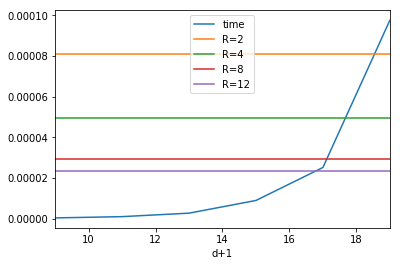In :
%matplotlib inline
import numpy as np
import pandas as pd

benchmarks_dir = 'https://www.di.ens.fr/~orru/hss/benchmarks/Intel.Core.i7-3537U/'
#benchmarks_dir = 'https://www.di.ens.fr/~orru/hss/benchmarks/Intel.Xeon.E5-2650/'

In :
ddlog = pd.read_csv(benchmarks_dir + 'ddlog.csv.gz', sep='\t', compression='gzip')
ddlog_ec17 = pd.read_csv(benchmarks_dir + 'ddlog-ec17.csv.gz', sep='\t', compression='gzip')

In :
# the average number of steps should be 2^failure - 2.
g = ddlog.groupby('d+1')
np.log2(g.mean())

Out:
steps time
d+1
9 8.980238 -21.130669
11 10.961174 -19.844769
13 12.988696 -18.458751
15 14.998823 -16.759804
17 16.999679 -15.274898
19 18.999671 -13.323055
21 21.000098 -11.579489
In :
# with a drawing:
plot = np.log2(g['steps'].mean()).plot()
plot.grid()
plot.set_ylabel('Steps (log2)')

Out:
<matplotlib.text.Text at 0x7fc4ce9d1da0>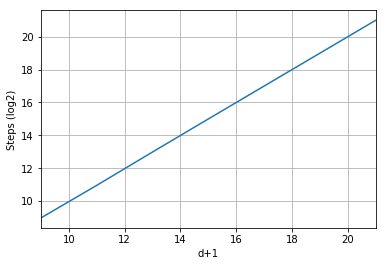In :
# and standard deviation should be roughly the same
np.log2(g.std())

Out:
steps time
d+1
9 8.945165 -20.830251
11 10.976854 -20.125113
13 12.969219 -18.522569
15 15.001511 -16.757033
17 17.001169 -15.217962
19 19.000940 -13.297938
21 21.000017 -11.570544
In :
# sanity check: do we have enough samples?
samples = ddlog.drop('time', axis=1).groupby('d+1')
samples.count() > samples.std() * 2.9

Out:
steps
d+1
9 True
11 True
13 True
15 True
17 True
19 True
21 True
In :
# what is the number of conversion steps for the different implementations of the conversion algorithm?
convsteps = pd.DataFrame({
'd+1': ddlog['d+1'],
'conversion steps': ddlog['time']/ddlog['steps'],
'conversion steps (ec17)': ddlog_ec17['time']/ddlog_ec17['steps'],
})
convsteps =1/convsteps.groupby('d+1').mean()[1:]
convsteps

Out:
conversion steps conversion steps (ec17)
d+1
11 1.575287e+09 1.320441e+09
13 2.598775e+09 1.770011e+09
15 3.376125e+09 2.918574e+09
17 4.969474e+09 3.742822e+09
19 5.268826e+09 4.109424e+09
21 6.357273e+09 5.025194e+09
In :
# so apparently you can't just multiply by the error probability to know the number of conversion steps.
convsteps.rename(columns={
'conversion steps':'Our implementation',
'conversion steps (ec17)':'Eurocrypt 2017 (w=64)'
}) \
.plot() \
.set_ylabel('conversion steps')

Out:
<matplotlib.text.Text at 0x7fc4cb121f98>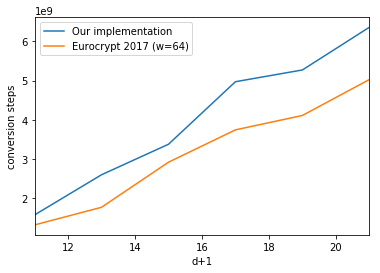In :
# using lookup tables we are able to get a factor of improvement of as much as:
a, b = convsteps['conversion steps (ec17)'], convsteps['conversion steps']
print('{:g}%'.format(max((b-a) / a) * 100))

46.8226%

In :
# how many conversion steps per second reached ?
print('{:g}'.format(max(convsteps['conversion steps'])))

6.35727e+09

In :
# assuming we are working with bits, and with a secret key basis B
# (thus M = B),
# what is the ratio of raised flags for different choices of basis B ?
flags = pd.DataFrame({
x : ddlog[ddlog['steps'] < 2**x].groupby('d+1').size() / ddlog.groupby('d+1').size() for x in range(2, 14, 2)
})
plot = np.log2(flags[4:]).plot()
plot.set_xlabel('d+1')
plot.set_ylabel('Raised Flag Frequency (log2)')
plot.legend(title='Secret Key base (log2)')

Out:
<matplotlib.legend.Legend at 0x7fc4c70a79e8>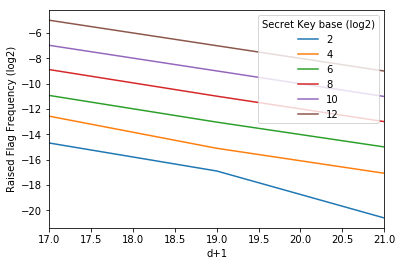In :
### Now let us look into group operations ###

In :
# what is the number of group operations per second
# knowing the time spent for computing 10^5 group operations?
group_operations = pd.read_csv(benchmarks_dir + 'group.csv', sep='\t')
group_operations = 1/group_operations.mean() * 10**5
group_operations

Out:
Z_p              1.029961e+06
Z_p (vanilla)    5.586734e+05
EC               4.106776e+06
dtype: float64
In :
# how much did we optimize wrt the vanilla algorithm?
group_operations['Z_p'] / group_operations['Z_p (vanilla)']

Out:
1.8435834198929768
In :
### Now let us look into exponentiations ###

In :
# As one would expect, the cost for a single exponentiation falls exponentially increasing "fb"
exponentiations = pd.read_csv(benchmarks_dir + 'fb_exp.csv', sep='\t')
plot = exponentiations.groupby('R').mean().plot()
plot.set_xlabel('Fixed Base (log2)')
plot.set_ylabel('Execution Time (s)')
plot.legend().remove()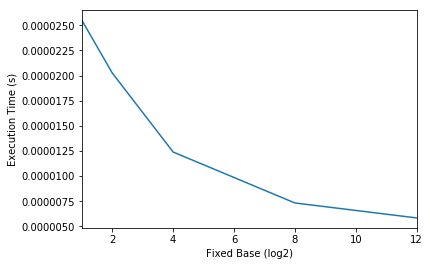In :
# sanity check: given that the exponent is 64-bits and thus we are doing 64/base multiplications, extrapolate mul/s
mult = group_operations['Z_p']
exp = exponentiations.groupby('R').mean()
# precomputation on x bits means that there are 64/x numbers to multiply, thus 64/x - 1 multiplications
exp =  1/ exp['time'] * (64/exp.index - 1)
exp.append(pd.DataFrame([mult])).rename({0: 'expected'}, columns={0: 'mult/s'})

Out:
mult/s
1 2.471402e+06
2 1.530834e+06
4 1.209882e+06
8 9.540814e+05
12 7.406648e+05
expected 1.029961e+06
In :
### Now let's look into full RMS. ###

In :
# how many RMS per second ?
rms = pd.read_csv(benchmarks_dir + 'rms.csv', sep='\t')
g = rms.groupby(['d+1', 'log2(B)', 'R']).mean()
1/g.rename(columns={'time': 'RMS/s'})

Out:
RMS/s
d+1 log2(B) R
17 1 1 53.753182
8 103.759842
12 117.458296
2 1 112.453304
8 250.635989
12 236.372533
4 1 206.387273
8 502.245035
In :
# with a drawing:
for failure_probability in (17, ):
table = rms[rms['d+1'] == failure_probability][['R', 'log2(B)', 'time']].groupby(['log2(B)', 'R']).mean()
plot = table.rename(columns={'time': 'time (s)'}).plot(kind='barh')
plot.set_title('Failure: {}'.format(failure_probability))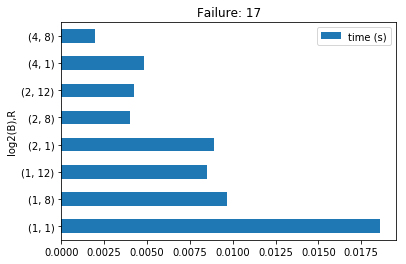In :
# sanity check: compare times for exponentiation + conversions with times for a full RMS.
# assume fb = 8, sb = 2, and d+1 = 17
fb, sb, dp1 = 8, 2, 17

conversion_times = ddlog.groupby('d+1').mean()['time']
exponentiation_times = exponentiations.groupby('R').mean()['time']

cnv_time = conversion_times[dp1]
exp_time = exponentiation_times[fb]
group_elements = 160 / sb + 1
# for every group element to process we have one conversion and 4 exponentiations.
time_expected = group_elements  * (cnv_time + exp_time * 4)
time_obtained = g['time'][dp1, sb, fb]
print('{:f}\t{:f}'.format(time_expected, time_obtained))

0.004420	0.003990

In :
# what is more relevant
p = conversion_times.to_frame()[:-1]
for fb in exponentiation_times.index[1:]:
p = p.assign(**{'R={}'.format(fb):  4*exponentiation_times[fb]})
p.plot()

Out:
<matplotlib.axes._subplots.AxesSubplot at 0x7fc4c701f2e8>